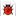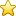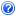# MATLAB智能算法30个案例分析.doc 108页

## 本文档一共被下载：次 ,您可全文免费在线阅读后下载本文档。

### 下载提示

1.本站不保证该用户上传的文档完整性，不预览、不比对内容而直接下载产生的反悔问题本站不予受理。
2.该文档所得收入(下载+内容+预览三)归上传者、原创者。
3.登录后可充值，立即自动返金币，充值渠道很便利文档纠错收藏文档下载帮助

• 内容提供方 晓风迷梦（上传创作收益人）
• 发布时间：2017-09-22
• 需要金币200(10金币=人民币1元)
• 浏览人气• 下载次数• 收藏次数• 文件大小：2 MB

MATLAB 智能算法30个案例分析 第 1 章 1、案例背景 遗传算法（Genetic Algorithm，GA）是一种进化算法，其基本原理是仿效生物界中的“物竞天择、适者生存”的演化法则。遗传算法的做法是把问题参数编码为染色体，再利用迭代的方式进行选择、交叉以及变异等运算来交换种群中染色体的信息，最终生成符合优化目标的染色体。 在遗传算法中，染色体对应的是数据或数组，通常是由一维的串结构数据来表示，串上各个位置对应基因的取值。基因组成的串就是染色体，或者叫基因型个体( Individuals)?。一定数量的个体组成了群体（Population)。群体中个体的数目称为群体大小（Population Size），也叫群体规模。而各个个体对环境的适应程度叫做适应度( Fitness)?。 1. 简单一元函数优化： clc clear all close all %% 画出函数图 figure(1); hold on; lb=1;ub=2; %函数自变量范围【1,2】 ezplot('sin(10*pi*X)/X',[lb,ub]); %画出函数曲线 xlabel('自变量/X') ylabel('函数值/Y') %% 定义遗传算法参数 NIND=40; %个体数目 MAXGEN=20; %最大遗传代数 PRECI=20; %变量的二进制位数 GGAP=0.95; %代沟 px=0.7; %交叉概率 pm=0.01; %变异概率 trace=zeros(2,MAXGEN); %寻优结果的初始值 FieldD=[PRECI;lb;ub;1;0;1;1]; %区域描述器 Chrom=crtbp(NIND,PRECI); %初始种群 %% 优化 gen=0; %代计数器 X=bs2rv(Chrom,FieldD); %计算初始种群的十进制转换 ObjV=sin(10*pi*X)./X; %计算目标函数值 while gen<MAXGEN FitnV=ranking(ObjV); %分配适应度值 SelCh=select('sus',Chrom,FitnV,GGAP); %选择 SelCh=recombin('xovsp',SelCh,px); %重组 SelCh=mut(SelCh,pm); %变异 X=bs2rv(SelCh,FieldD); %子代个体的十进制转换 ObjVSel=sin(10*pi*X)./X; %计算子代的目标函数值 [Chrom,ObjV]=reins(Chrom,SelCh,1,1,ObjV,ObjVSel); %重插入子代到父代，得到新种群 X=bs2rv(Chrom,FieldD); gen=gen+1; %代计数器增加 %获取每代的最优解及其序号，Y为最优解,I为个体的序号 [Y,I]=min(ObjV); trace(1,gen)=X(I); %记下每代的最优值 trace(2,gen)=Y; %记下每代的最优值 end plot(trace(1,:),trace(2,:),'bo'); %画出每代的最优点 grid on; plot(X,ObjV,'b*'); %画出最后一代的种群 hold off %% 画进化图 figure(2); plot(1:MAXGEN,trace(2,:)); grid on xlabel('遗传代数') ylabel('解的变化') title('进化过程') bestY=trace(2,end); bestX=trace(1,end); fprintf(['最优解:\nX=',num2str(bestX),'\nY=',num2str(bestY),'\n']) 2. 多元函数优化 clc clear all close all %% 画出函数图 figure(1); lbx=-2;ubx=2; %函数自变量x范围【-2,2】 lby=-2;uby=2; %函数自变量y范围【-2,2】 ezmesh('y*sin(2*pi*x)+x*cos(2*pi*y)',[lbx,ubx,lby,uby],50); %画出函数曲线 hold on; %% 定义遗传算法参数 NIND=40; %个体数目 MAXGEN=50; %最大遗传代数 PRECI=20; %变量的二进制位数 GGAP=0.95; %代沟 px=0.7; %交叉概率 pm=0.01; %变异概率 trace=zeros(3,MAXGEN); %寻优结果的初始值 FieldD=[PRECI PRECI;lbx lby;ubx uby;1 1;0 0;1 1;1 1]; %区域描述器 Chrom=crtbp(NIND,PRECI*2); %初始种群 %% 优化 gen=0; %代计数器 XY=bs2rv(Ch

“原创力文档”前称为“文档投稿赚钱网”，本站为“文档C2C交易模式”，即用户上传的文档直接卖给(下载)用户，本站只是中间服务平台，本站所有文档下载所得的收益归上传人(含作者)所有【成交的100%(原创)】。原创力文档是网络服务平台方，若您的权利被侵害，侵权客服QQ：3005833200 电话：19940600175 欢迎举报，上传者QQ群:784321556